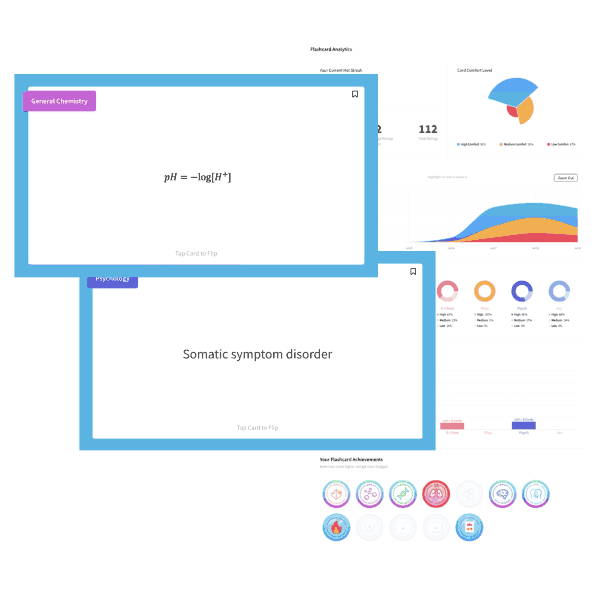# MCAT Chemistry Question — Catalyst

A scientist adds a small amount of catalyst to a 1 L reaction vessel containing 2.03 mol of A2, 6.89 mol of B3C, 1.40 mol of AB2, and 2.50 mol of C2. Which of the following will occur?

3 A2 (g) + 4 B3C (g) → 6 AB2 (g) + 2 C2 (g)

Keq = 5.13 x 102

A. The reaction will more swiftly proceed to the left.

B. The reaction will more swiftly proceed to the right.

C. The addition of a catalyst does nothing to a reaction at equilibrium, so the reaction will remain at equilibrium.

D. No conclusion can be drawn.

##### Explanation

Begin by setting up the reaction quotient equation for this reaction:

Q = ([AB2]6[C2]2) / ([A2]3[B3C]4)

Then plug in the values given:

Q = (1.46 x 2.52) / (2.033 x 6.894)

The numbers are tough to deal with, so we’ll start by rounding them off:

Q = (1.56 x 2.52) / (23 x 74)

Notice that we made the numerator bigger by rounding 1.4 up, so we need to make the denominator bigger by rounding up to 7. As much as possible, make your approximations cancel each other out.

We’ll still want to chip away at this by breaking down the numbers into small pieces:

Q = ((1.52)(1.52)(1.52)x 2.52) / (23 x (72)(72))

Q = ((2.25)(2.25)(2.25)x 6.25) / (8 x (49)(49))

Round the 49 up to 50:

Q = ((2.25)(2.25)(2.25)x 6.25) / (8 x (50)(50))

Q = ((2.25)(2.25)(2.25)x 6.25) / (8 x (2,500))

Q = ((2.25)(2.25)(2.25)x 6.25) / (20,000)

Round the 2.25 down to 2 and the 6.25 up to 7 (remember, make your approximations cancel each other out):

Q = (2 x 2 x 2 x 7) / (20,000)

Q = (8 x 7) / (20,000)

Q = 56 / 20,000

Express in scientific notation:

Q = (5.6 x 101) / (2.0 x 104)

Q = (5.6 / 2) x (101 / 104)

Q = 2.8 x 10-3

Now that we’ve approximated Q, we can compare it to Keq to see what will happen. Here, the Q is nearly 100,000 times smaller than the Keq. That means our reaction quotient has far too many reactants (bigger denominator means a smaller number). So the reaction will move towards products, to the right.

Note that the arithmetic in this question is more difficult than you will likely see on the MCAT, simply due to the number of steps involved. The key lesson from this question, though, is that the MCAT absolutely will expect you to be able to approximate, and will expect you to solve questions that compare Q and Keq.

An alternate way to deal with this question would be simply to look at the sizes of the numbers involved. That is, you see a 74 in the denominator. You could look at that and guess “okay this fraction will be less than 1. I don’t know how much less, but probably less than 1.” Then compare that to the Keq, which is around 500, and arrive at answer (B).

## Want more MCAT practice?

### We’ve got options for every schedule and learning style!

From the best online MCAT course created by top instructors with 524+ MCAT scores to the most representative full-length practice exams and private tutoring, we can custom tailor your MCAT prep to your goals!

Not sure which option is right for you? Schedule a free MCAT consultation with an MCAT expert using the form below. No obligation, just expert advice.

Create your Free Account to access our MCAT FlashcardsMCAT is a registered trademark of the Association of American Medical Colleges (AAMC), which is not affiliated with Blueprint.